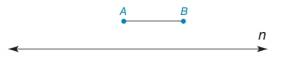Chapter 1.3, Problem 32E### Elementary Geometry for College St...

6th Edition
Daniel C. Alexander + 1 other
ISBN: 9781285195698

#### Solutions

Chapter
Section### Elementary Geometry for College St...

6th Edition
Daniel C. Alexander + 1 other
ISBN: 9781285195698
Textbook Problem
1 views

# In Exercises 29 to 32, use only a compass and a straightedge to complete each construction.Exercises 31, 32 Given: A B ¯ as shown in the figure Construct: T V ¯ on line n so that T V = 1 2 ( A B )

To determine

To find:

The complete construction of TV¯ on line n so that TV=12(AB).

Explanation

Given:

The given figure is,

Approach:

The steps are given below for the complete construction.

a) Draw a straight line and label a point T.

b) Use compass and open it to the width of AB¯. Use T as center, mark the measured distance and label the point as X.

c) Use T and X as the centers for the compass and take the radius greater than the length of 12AB.

d) These arcs intersect above and below the line.

e) Join the intersections of the arcs

### Still sussing out bartleby?

Check out a sample textbook solution.

See a sample solution

#### The Solution to Your Study Problems

Bartleby provides explanations to thousands of textbook problems written by our experts, many with advanced degrees!

Get Started

#### Find more solutions based on key concepts